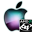ATOMS : krisp details

# krisp

Kriging based regression and optimization package for Scilab.
Details
Version
0.95
A more recent valid version with binaries for Scilab 5.3 exists: 0.96
Author
Janis Janusevskis
Owner Organization
EMSE, CROCUS
Maintainer
Janis Janusevskis
Category
Dependency
Creation Date
January 3, 2012
Source created on
Scilab 5.3.x
Binaries available on
Scilab 5.3.x:Linux 32-bitWindows 32-bitWindows 64-bitmacOSLinux 64-bit
Install command
`--> atomsInstall("krisp")`
Report a bugDescription
```            KRIging Scilab Package

Kriging based regression and optimization package for Scilab.

This toolbox implements kriging based regression (also known as Gaussian process
regression) and optimization of deterministic simulators.
The toolbox consists of two main components:
1. functions for creation of kriging model for deterministic or noisy data
(correlation kernels, hyper-parameter estimation, prediction, cross
validation).
2. methods implementing Efficient Global Optimization (EGO) for time consuming
deterministic functions.

Included trend functions: constant, linear, quadratic polynomials.
Included correlation kernels: Gaussian, Matern_5_2, power exponential (p=1.9)
and exponential (p=1);

For sub-optimization (kriging hyper-parameter learning and EI maximization) use
CMA-ES (v0.955) (http://www.lri.fr/~hansen/cmaes_inmatlab.html) or
//-----------------------------
The main functions are:

macros/Kriging/ 	- functions for creation of kriging model.

km - create kriging model.
findtheta - find kriging hyper-parameters.
predict - calculate prediction of kriging mean and variance.
LOOCV - calculate Leave One Out Cross-validation given by kriging model.
logLL - calculate negative log likelihood of kriging model.

simGaussian  - simulate Gaussian processes based on covariance and trend
information.
SimCondGaussian - simulate conditional Gaussian processes based on kriging
model.

macors/EGO/ 	- functions for optimization of deterministic simulator based on
kriging model.

EGO- create EGO optimizer.
EGO_ask - get next data points.
EGO_tell - to tell optimizer response values at new data points.
EGO_stop - indicates if stopping criterion has been reached.
EGO_best - returns the best point and criterion value.

src/c/
sci_gateway/c/
- C functions for correlation matrix and correlation vector creation.

For computational efficiency correlation matrix and vector creation is
implemented in C.
See:
CorrMatrix.sci and sci_corr_D.c 	-	for creation of kriging correlation
matrix.
CorrVectorData.sci and sci_corr_vector.c	-	 for creation of correlation vector
between point and observed data points.
createCorr.sci and sci_corr_X.c	-	 for creation of arbitrary correlation
matrix.
corr_functions.h	-	 macros of correlation kernels.

*krisp_0.95
Correlation matrix and vector creation implemented using C gateways and Scilab
API.
Demos modified.
km.sci modified: input parameters, kmodel structure.
findTheta.sci modified: CMA default with restarts; optimization settings are
saved into kmodel structure.
ego.sci modified: input parameters, EGO structure does not contain kriging
parameter estimation settings any more.
EGO_ask.sci modified: CMA default  with restarts.
EGO_tell.sci modified: findTheta is called using optimization settings in
kmodel structure.
some errors in help files corrected.

```Files (6)Source code archive
```
```Linux 32-bit binary for Scilab 5.3.x
```Linux 32-bit
Automatically generated by the ATOMS compilation chain

```Windows 32-bit binary for Scilab 5.3.x
```Windows 32-bit
Automatically generated by the ATOMS compilation chain

```Windows 64-bit binary for Scilab 5.3.x
```Windows 64-bit
Automatically generated by the ATOMS compilation chain

```macOS binary for Scilab 5.3.x
```MacOSX version
Automatically generated by the ATOMS compilation chain

```Linux 64-bit binary for Scilab 5.3.x
```Linux 64-bit
Automatically generated by the ATOMS compilation chain

```News (0)Comments (0)Leave a comment
Login withEmail notifications